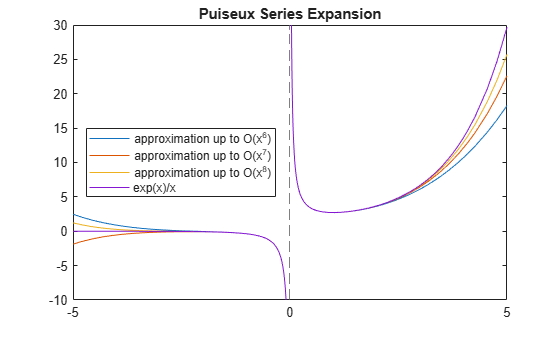# series

Puiseux series

## Syntax

``series(f,var)``
``series(f,var,a)``
``series(___,Name,Value)``

## Description

example

````series(f,var)` approximates `f` with the Puiseux series expansion of `f` up to the fifth order at the point `var = 0`. If you do not specify `var`, then `series` uses the default variable determined by `symvar(f,1)`.```

example

````series(f,var,a)` approximates `f` with the Puiseux series expansion of `f` at the point ```var = a```.```

example

````series(___,Name,Value)` uses additional options specified by one or more `Name,Value` pair arguments. You can specify `Name,Value` after the input arguments in any of the previous syntaxes.```

## Examples

### Find Puiseux Series Expansion

Find the Puiseux series expansions of univariate and multivariate expressions.

Find the Puiseux series expansion of this expression at the point `x = 0`.

```syms x series(1/sin(x), x)```
```ans = x/6 + 1/x + (7*x^3)/360```

Find the Puiseux series expansion of this multivariate expression. If you do not specify the expansion variable, `series` uses the default variable determined by `symvar(f,1)`.

```syms s t f = sin(s)/sin(t); symvar(f, 1) series(f)```
```ans = t ans = sin(s)/t + (7*t^3*sin(s))/360 + (t*sin(s))/6```

To use another expansion variable, specify it explicitly.

```syms s t f = sin(s)/sin(t); series(f, s)```
```ans = s^5/(120*sin(t)) - s^3/(6*sin(t)) + s/sin(t)```

### Specify Expansion Point

Find the Puiseux series expansion of `psi(x)` around ```x = Inf```. The default expansion point is 0. To specify a different expansion point, use the `ExpansionPoint` name-value pair.

`series(psi(x), x, 'ExpansionPoint', Inf)`
```ans = log(x) - 1/(2*x) - 1/(12*x^2) + 1/(120*x^4)```

Alternatively, specify the expansion point as the third argument of `series`.

```syms x series(psi(x), x, Inf)```
```ans = log(x) - 1/(2*x) - 1/(12*x^2) + 1/(120*x^4)```

### Plot Puiseux Series Approximation

Find the Puiseux series expansion of `exp(x)/x` using different truncation orders.

Find the series expansion up to the default truncation order 6.

```syms x f = exp(x)/x; s6 = series(f, x)```
```s6 =  $\frac{x}{2}+\frac{1}{x}+\frac{{x}^{2}}{6}+\frac{{x}^{3}}{24}+\frac{{x}^{4}}{120}+1$```

Use `Order` to control the truncation order. For example, approximate the same expression up to the orders 7 and 8.

`s7 = series(f, x, 'Order', 7)`
```s7 =  $\frac{x}{2}+\frac{1}{x}+\frac{{x}^{2}}{6}+\frac{{x}^{3}}{24}+\frac{{x}^{4}}{120}+\frac{{x}^{5}}{720}+1$```
`s8 = series(f, x, 'Order', 8)`
```s8 =  $\frac{x}{2}+\frac{1}{x}+\frac{{x}^{2}}{6}+\frac{{x}^{3}}{24}+\frac{{x}^{4}}{120}+\frac{{x}^{5}}{720}+\frac{{x}^{6}}{5040}+1$```

Plot the original expression `f` and its approximations `s6`, `s7`, and `s8`. Note how the accuracy of the approximation depends on the truncation order.

```fplot([s6 s7 s8 f]) legend('approximation up to O(x^6)','approximation up to O(x^7)',... 'approximation up to O(x^8)','exp(x)/x','Location', 'Best') title('Puiseux Series Expansion')```### Specify Direction of Expansion

Find the Puiseux series approximations using the `Direction` argument. This argument lets you change the convergence area, which is the area where `series` tries to find converging Puiseux series expansion approximating the original expression.

Find the Puiseux series approximation of this expression. By default, `series` finds the approximation that is valid in a small open circle in the complex plane around the expansion point.

```syms x series(sin(sqrt(-x)), x)```
```ans = (-x)^(1/2) - (-x)^(3/2)/6 + (-x)^(5/2)/120```

Find the Puiseux series approximation of the same expression that is valid in a small interval to the left of the expansion point. Then, find an approximation that is valid in a small interval to the right of the expansion point.

```syms x series(sin(sqrt(-x)), x) series(sin(sqrt(-x)), x, 'Direction', 'left') series(sin(sqrt(-x)), x, 'Direction', 'right')```
```ans = (-x)^(1/2) - (-x)^(3/2)/6 + (-x)^(5/2)/120 ans = - x^(1/2)*1i - (x^(3/2)*1i)/6 - (x^(5/2)*1i)/120 ans = x^(1/2)*1i + (x^(3/2)*1i)/6 + (x^(5/2)*1i)/120```

Try computing the Puiseux series approximation of this expression. By default, `series` tries to find an approximation that is valid in the complex plane around the expansion point. For this expression, such approximation does not exist.

`series(real(sin(x)), x)`
```Error using sym/series>scalarSeries (line 90) Unable to compute series expansion.```

However, the approximation exists along the real axis, to both sides of `x = 0`.

`series(real(sin(x)), x, 'Direction', 'realAxis')`
```ans = x^5/120 - x^3/6 + x```

## Input Arguments

collapse all

Input to approximate, specified as a symbolic expression or function. It also can be a vector, matrix, or multidimensional array of symbolic expressions or functions.

Expansion variable, specified as a symbolic variable. If you do not specify `var`, then `series` uses the default variable determined by `symvar(f,1)`.

Expansion point, specified as a number, or a symbolic number, variable, function, or expression. The expansion point cannot depend on the expansion variable.

You also can specify the expansion point as a `Name,Value` pair argument. If you specify the expansion point both ways, then the `Name,Value` pair argument takes precedence.

### Name-Value Arguments

Specify optional comma-separated pairs of `Name,Value` arguments. `Name` is the argument name and `Value` is the corresponding value. `Name` must appear inside quotes. You can specify several name and value pair arguments in any order as `Name1,Value1,...,NameN,ValueN`.

Example: `series(psi(x),x,'ExpansionPoint',Inf,'Order',9)`

Expansion point, specified as a number, or a symbolic number, variable, function, or expression. The expansion point cannot depend on the expansion variable.

You can also specify the expansion point using the input argument `a`. If you specify the expansion point both ways, then the `Name,Value` pair argument takes precedence.

Truncation order of Puiseux series expansion, specified as a positive integer or a symbolic positive integer.

`series` computes the Puiseux series approximation with the order `n - 1`. The truncation order `n` is the exponent in the O-term: O(varn).

Direction for area of convergence of Puiseux series expansion, specified as:

 `'left'` Find a Puiseux series approximation that is valid in a small interval to the left of the expansion point. `'right'` Find a Puiseux series approximation that is valid in a small interval to the right of the expansion point. `'realAxis'` Find a Puiseux series approximation that is valid in a small interval on the both sides of the expansion point. `'complexPlane'` Find a Puiseux series approximation that is valid in a small open circle in the complex plane around the expansion point. This is the default value.

## Tips

• If you use both the third argument `a` and the `ExpansionPoint` name-value pair to specify the expansion point, the value specified via `ExpansionPoint` prevails.